## Pages

`“Life is like riding a bicycle.  To keep your balance you must keep moving.”–Albert Einstein`

## Saturday, June 27, 2009

### AP Physics B – Answer to Free Response Practice Question involving Friction

In the post dated 25th June 2009, a free-response practice question involving friction was given for the benefit of AP Physics B aspirants. As promised, I give below the answer along with the question:The figure (Fig.1) shows a block of mass M, placed on a plane surface AB inclined at an angle θ with the horizontal AC. Answer the questions relevant to the situations given below:

(a) Initially the frictional force Ff between the inclined plane and the block is sufficient to keep the block at rest. The coefficient of static friction between the incline and the block is μ. On the dot below (Fig.3) that represents the block, draw and label a free body diagram for the block in this situation.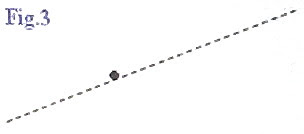(b) Determine the magnitude of the frictional force on the block in the situation described in part (a).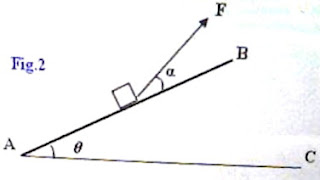(c) A force is now applied on the block in a direction making an angle α (Fig.2) with the inclined plane so that the block just moves up the plane.

Determine the magnitude of the minimum force (Fmin) required for pulling the block up the plane.

(d) The inclination of the plane AB with the horizontal is made equal to 30º. When a block of mass 2 kg is placed on the incline and a gentle push is given, the block moves down the plane with constant velocity of 0.2 ms–1.

Determine the magnitude of the frictional force on the block and the coefficient of friction in this situation, assuming that g = 10 ms–2.

(a) The free body diagram is shown in Fig.4 in which Mg is the weight of the block, N is the normal force exerted on the block (by the inclined plane) and Ff is the frictional force on the block. The frictional force prevents the block from sliding down.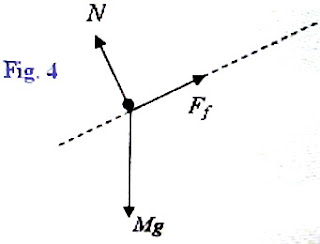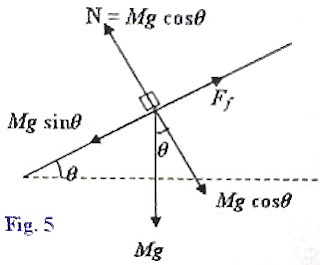(b) The forces acting on the block are shown in the adjoining figure (Fig.5). The component (Mgsinθ) of the weight of the body, parallel to the incline is the force trying to move the body down the plane. The normal force N is the reaction (force) opposing the component Mgcosθ of the weight of the body normal to the inclined plane. The frictional force Ff is opposite to the component Mg sinθ parallel to the plane. Since the block is at rest, the net force acting on it is zero and Ff is just sufficient to balance the component Mg sinθ (of the weight of the body).

Thus the magnitude of the frictional force on the block in this situation is Mg sinθ.

(c) When a force F is applied in the direction α as given in the question (Fig. 2), the component of this force normal to the inclined plane (Fsinα) opposes the component Mgcosθ of the weight of the body. The normal force pressing the inclined plane therefore is equal to Mgcosθ F sinα. The normal reaction (or, normal force) exerted by the inclined plane on the block is therefore given in this case by

N = Mgcosθ F sinα

The frictional force opposing the motion of the block upwards is μN = μ(Mgcosθ F sinα).

When the block just begins to move up the incline, the net force acting on it is zero. The force component trying to pull the block up the incline is Fcosα and the forces trying to oppose the motion are the weight component Mgsinθ and the frictional force = μ(Mgcosθ F sinα).

Therefore, we have Fcosα = Mgsinθ + μ(Mgcosθ F sinα)

Or, F = Mg(sinθ + μcosθ) /(cosα + μ sinα)

Since μ = tan λ where λ is the angle of friction, the above equation can be rewritten as

F = Mg [sinθ + (sinλ/cosλ) cosθ)] /[(cosα + (sinλ/cosλ) sinα)]

= Mg(sinθ cosλ + cosθ sinλ) /(cosα cosλ + sinα sinλ)

Or, F = Mg sin(θ + λ) /cos(α λ)

The pulling force will be minimum when the denominator cos(α λ) is maximum, equal to 1.

Thus the magnitude of the minimum force (Fmin) required for pulling the block up the plane is given by

Fmin = Mg sin(θ + λ)

[Note that cos(α λ) = 1 when α = λ. So the inclination of the pulling force with the inclined plane must be equal to the angle of friction].

(d) Since the block is moving down with uniform velocity, the net force acting on the block is zero. In this situation the frictional force is μMgcosθ and it acts up the plane, opposing the downward motion of the block. The component of the weight Mgsinθ of the body acts down the plane and tries to drive the body down the plane. These are thus equal in magnitude and opposite in direction.

[The velocity 0.2 ms–1 mentioned in the question should not distract you. It does not affect the answer as long as it is uniform. If its magnitude is smaller than the given value, it just means that the initial push given to the block was softer].

The magnitude of the frictional force in this situation is thus given by

Ff = Mgsinθ = 2×10× sin 30º = 10 newton.

Since μMgcosθ = Mgsinθ, the coefficient of friction is given by

μ = tanθ = tan 30º = 0.5774

You will find some useful multiple choice questions involving friction at physicsplus

## Thursday, June 25, 2009

### AP Physics B – Free Response Practice Question involving Friction

Free response as well as multiple choice questions involving friction are often seen in degree entrance examinations. Friction is a common force with interesting properties and it plays a very important role in our life. For instance see the post Friction Moves the Car and Friction Stops the Car at Physicsplus.The figure (Fig.1) shows a block of mass M, placed on a plane surface AB inclined at an angle θ with the horizontal AC. Answer the questions relevant to the situations given below:

(a) Initially the frictional force Ff between the inclined plane and the block is sufficient to keep the block at rest. The coefficient of static friction between the incline and the block is μ. On the dot below (Fig.3)that represents the block, draw and label a free body diagram for the block in this situation.

(b) Determine the magnitude of the frictional force on the block in the situation described in part (a).(c) A force F is now applied on the block in a direction making an angle α (Fig.2) with the inclined plane so that the block just moves up the plane.

Determine the magnitude of the minimum force (Fmin) required for pulling the block up the plane.

(d) The inclination of the plane AB with the horizontal is made equal to 30º. When a block of mass 2 kg is placed on the incline and a gentle push is given, the block moves down the plane with constant velocity of 0.2 ms–1.

Determine the magnitude of the frictional force on the block and the coefficient of friction in this situation, assuming that g = 10 ms–2.

Even though this question is meant for AP Physics B students, AP Physics C aspirants also may work it out. The question can be easily modified to suit to the AP Physics C students.

Try to answer the above question which carries 15 points. You can take about 17 minutes for answering it. I’ll be back shortly with a model answer for you.

## Thursday, June 18, 2009

### AP Physics B&C – Answer to Free Response Practice Question on Newton’s Laws

In the post dated 15th June 2009, the following free-response question for practice was given to you: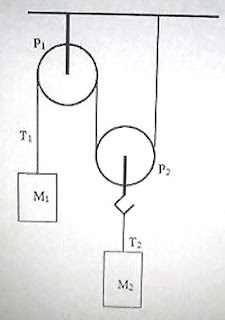In the adjoining figure P1 and P2 are light frictionless pulleys. P1 is fixed and P2 is movable. The strings used are inextensible and of negligible mass. The fixed pulley P1 carries a mass M1 and the movable pulley P2 carries a mass M2 which has one and a half times the mass of M1. Now, answer the following questions:

(a) How is the tension T1 in the string attached to the mass M1 related to the tension T2 in the string attached to the mass M2?

(b) How is the magnitude of acceleration a1 of the mass M1 related to the magnitude of acceleration a2 of the mass M2?

(c) From the following options regarding the motion of the mass M1, select the correct one by putting a tick (√) mark:

Mass M1 will be accelerated upwards ______

Mass M1 will be accelerated downwards ______

Mass M1 will move with uniform velocity ______

(d) Determine the accelerations a1 and a2 in of the masses M1 and M2 respectively.

(e) Determine the tensions T1 and T2 in the strings attached to the masses M1 and M2 respectively.

(a) The forces acting on the system of masses are shown in the figure. The string passing over the pulleys P1 and P2 has the same tension T1 everywhere. Since the tension T2 in the string connected to the mass M2 is produced because of the tensions T1 and T1 it follows that

T2 = T1 + T1 = 2 T1

(b) When the mass M1 moves through a distance x, the mass M2 moves through a distance x/2 only. Therefore the magnitude of acceleration a1 of the mass M1 is twice the magnitude of acceleration a2 of the mass M2:

a1 = 2a2

(c) If the masses were such that the system were stationary we would have

M1g = T1 and M2g = T2 = 2 T1

From the above equations M2 = 2M1.

Since it is given in the question that M2 = 1.5 M1, it follows that the system cannot be stationary. Evidently the mass M1 will be accelerated downwards (and M2 will be accelerated upwards).

(d) The net force acting on M1 = M1g T1

Therefore, M1g T1 = M1a1 ………………(i)

Similarly, considering mass M2 we obtain

2T1 M2g = M2 a1/2 …………….(ii)

[We have used T2 = 2T1 and a2 = a1/2].

Multiplying Eq.(i) by 2 and adding to Eq.(ii) we obtain

2M1g M2g = a1[2M1 + (M2/2)]

This gives

a1 = 2g(2M1M2) /(4M1 + M2)

This is directed downwards.

Since a2 = a1/2 we have

a2 = g(2M1M2) /(4M1 + M2), which is directed upwards.

(e) Substituting for a1 in Eq.(i),

T1 = M1(g a1) = M1[g {2g(2M1M2) /(4M1 + M2)}]

Or, T1 = M1[(4M1 + M2)g 2g(2M1M2)] /(4M1 + M2)

This simplifies to T1 = 3M1M2g /(4M1 + M2)

Since T2 = 2T1 we obtain

T2 = 6M1M2g /(4M1 + M2)

## Monday, June 15, 2009

### AP Physics B&C – Free Response Practice Question on Newton’s Laws

The following question will be useful for AP Physics B as well as AP Physics C aspiramts. Parts (a), (b), (c) and (d) of the question are meant for B as well as C. Part (d) of the question is meant additionally for AP physics C aspirants. Here is the question:In the adjoining figure P1 and P2 are light frictionless pulleys. P1 is fixed and P2 is movable. The strings used are inextensible and of negligible mass. The fixed pulley P1 carries a mass M1 and the movable pulley P2 carries a mass M2 which has one and a half times the mass of M1. Now, answer the following questions:

(a) How is the tension T1 in the string attached to the mass M1 related to the tension T2 in the string attached to the mass M2?

(b) How is the magnitude of acceleration a1 of the mass M1 related to the magnitude of acceleration a2 of the mass M2?

(c) From the following options regarding the motion of the mass M1, select the correct one by putting a tick (√) mark:

Mass M1 will be accelerated upwards ______

Mass M1 will be accelerated downwards ______

Mass M1 will move with uniform velocity ______

(d) Determine the accelerations a1 and a2 of the masses M1 and M2 respectively.

(e) Determine the tensions T1 and T2 in the strings attached to the masses M1 and M2 respectively.

Try to answer the above question. I’ll be back soon with a model answer for your benefit.

You can access all posts related to Newton’s laws of motion on this site by clicking on the label ‘Newton’s laws’ below this post.

## Friday, June 12, 2009

### AP Physics B & C- Try to Find Answers to Old Questions

-->
Your preparation for any examination will be incomplete if you do not work out a reasonably large number of old questions. With the universal use of the internet, the present day students are at a great advantage since it is a common practice for exam conducting bodies to publish old questions on their web sites.
I have often come across queries from AP Physics aspirants regarding the sites from where they can get old AP Physics questions. Fortunately for them (and of course for AP Teachers as well) the College Board has made it a practice to publish the Free Response questions in respect of earlier AP Physics examinations. The scoring guidelines also are published on their web site. Very useful details are given in the scoring guidelines so that the students will be careful to make their presentation fool proof when they take up their exam. Home schooled students as well as students whose schools do not offer AP courses will be particularly benefited by the questions and scoring guidelines published by the College Board.
The following links will take you to the locations where you will find the old questions and scoring guidelines in respect of AP Physics B and AP Physics C examinations respectively:
(i) AP Physics B Free Response Questions & Scoring Guidelines:
(ii) AP Physics C (Electricity & Magnetism) Free Response Questions & Scoring Guidelines
(iii) AP Physics C (Mehanics) Free Response Questions & Scoring Guidelines:
https://apstudent.collegeboard.org/apcourse/ap-physics-c-mechanics/exam-practice
You should understand that older question papers can serve as a useful tool in your endeavour. Physics is a subject with vast scope for designing questions in numerous ways to gauge your knowledge, understanding and capacity for application of any concept. So try to master the subject, taking into account the prescribed syllabus.

## Sunday, June 7, 2009

### AP Physics B & C Kinematics- More Multiple Choice Questions on One Dimensional Motion

The best thinking has been done in solitude. The worst has been done in turmoil

– Thomas Alva Edison

The essential things you need to remember in one dimensional uniformly accelerated motion were discussed in the post dated 13th August 2008. A few multiple choice questions on one dimensional motion were discussed in the post dated 21st August 2008. You can access both posts by clicking on the label ‘one dimensional motion’ below this post. Today we will discuss a few more multiple choice practice questions in this section: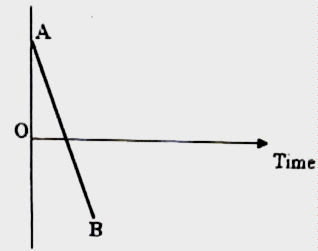(1) In the straight line graph AB shown in the adjoining figure time is taken along the x-axis. The quantity taken along the y-axis (which is not shown) in the graph can be

(a) acceleration of a train arriving at a railway station

(b) velocity of a train arriving at a railway station

(c) velocity of a cricket ball thrown towards a batsman

(d) acceleration of a ball thrown vertically downwards

(e) velocity of a ball thrown vertically upwards

When a ball is thrown vertically upwards, its velocity v goes on decreasing linearly with time t in accordance with the equation,

v = v0 gt where v0 is the velocity of projection and g is the acceleration due to gravity.

At the maximum height the velocity is zero. Thereafter the direction of the velocity is reversed (during the return trip of the ball) and the magnitude of velocity goes on increasing linearly with time in accordance with the equation,

v = v0 + gt

Therefore, the graph AB is the velocity-time graph of a body projected vertically upwards. Option (e) is correct.

(2) An iron sphere released from rest at a height h falls freely under gravity. It reaches half the height at the instant t1 and hits the ground at the instant t2. if air resistance is negligible, the ratio t1/t2 is

(a) 21/2

(b) 2–1/2

(c) 2–1/4

(d) 21/4

(e) ¼

We have s = v0 t+ (½) at2 where s is the displacement in time t, v0 is the initial velocity and a is the acceleration.

Since the displacements in times t1 and t2 are h/2 and h respectively, we have

h/2 = 0 + (½) g t12 and

h= 0 + (½) g t22

Therefore t12/t22 = ½ so that t1/t2 = 2–1/2

(3) A rifle can fire bullets with a speed of 1000 ms–1. Assume that air resistance is negligible and the gravitational acceleration is 10 ms–2. In order to hit a target at a horizontal distance of 100 m, the rifle is to be aimed

(a) 5 cm above the target

(b) 10 cm above the target

(c) 10 cm below the target

(d) 5 cm below the target

(e) directly at the target

Since the gravitational force does not affect the horizontal velocity of the bullet, the time t taken to cover the horizontal distance of the target is given by

t = 100/1000 = 0.1 s

During this time the bullet will fall down by a distance s given by

s = ½ gt2 = ½ ×10×(0.1)2 = 0.05 m = 5 cm.

Therefore, in order to hit the target, the rifle is to be aimed at a point 5 cm above the target [Option (a)].

(4) When a body is thrown vertically down with a velocity of 4 ms–1 from a height h it reaches the ground with a velocity of 5 ms–1. If the same body is is released from rest from the same height h, it it will reach the ground with a velocity

(a) 2 ms–1

(b) 2.5 ms–1

(c) 3 ms–1

(d) 3.5 ms–1

(e) 4 ms–1

We can use here the equation of uniformly accelerated motion,

v2 = u2 + 2as where u is the initial velocity and v is the final velocity after a body moving with an acceleration a suffers a displacement s.

When the body is thrown down with a velocity of 4 ms–1 from a height h we have

52 = 42 + 2gh …….(i)

where g is the acceleration due to gravity.

When the body is released from rest from the same height h we have

v2 = 0 + 2gh ……..(ii)

Subtracting Eq.(ii) from Eq.(i) we obtain

52 v2 = 42 from which v = 3 ms–1.

The following questions [(5) and (6)] are meant for AP Physics C aspirants:

(5) A particle moving along the positive x-direction has its position coordinate x at time t given by

x = b t+ c where b and c are constants.

The acceleration and the initial velocity of the particle are respectively

(a) zero, b

(b) zero, 2bc

(c) b2, bc

(d) b2, 2bc

(e) 2b2, 2bc

Squaring the equation for the position coordinate, we have

x = b2t2 + c2 + 2bct

The velocity v of the particle at the instant t is given by

v = dx/dt = 2 b2t + 2bc

Or, v =2bc + 2b2t

When t = 0, v = 2bc. This is the initial velocity of the particle.

The acceleration a of the particle is given by

a = dv/dt = 2b2

[You can obtain the answers from the form of the equation, x = b2t2 + c2 + 2bct itself. This equation is in the form, x = x0 + v0t + ½ at2 which gives the displacement (x x0) at the instant t of a particle moving along a straight line with acceleration a and initial velocity v0].

(6) A ball projected vertically up from the ground moves past a point P at a height h at the end of 2 seconds during the upward trip. After another 2 seconds it moves past the same point P during the downward trip. Neglect air resistance and assume that g = 10 ms–2. The maximum height reached by the ball is

(a) 50 m

(b) 45 m

(c) 40 m

(d) 33 m

(e) 22 m

The time taken by the ball to reach the maximum height is 2 +1 = 3 s.

If u is the velocity of projection and t is the time taken to reach the maximum height, we have (from the equation v = u + at)

0 = u gt = u – 10×3

This gives u = 30 ms–1

The maximum height reached is ut – ½ gt2 = 30×3 – ½ ×10×32 = 45 m.

(7) A car starts from rest and moves along straight road with uniform acceleration α for some time. Then it moves with uniform retardation β and comes to rest. If the total time of travel is t what is the maximum velocity attained by the car?

(a) 2αβt /(α+ β )

(b) (α+ β )tβ

(c) αβt /(α+ β )

(d) αt /(α+ β )

(e) α2βt /(α+ β )

If t1 is the time interval during which the car was accelerated and v is the maximum velocity of the car during the trip we have the following equations considering the accelerated and decelerated parts of the trip respectively:

v =0 + α t1 …………….(i)

0 = v β(t t1) ………..(ii)

From these equations, α t1 = β(t t1).

Or, t1(α+ β ) = βt from which t1 = βt /(α+ β ).

From Eq.(i) the maximum velocity v = α t1.

Substituting for t1, the maximum velocity v = αβt /(α+ β ).

(8) In the above question what is the distance traveled during the decelerated part of the trip?

(a) αβ t2 /2(α+ β )2

(b) αβ t2 /2(α+ β )

(c) α2β t2 /2(α+ β )2

(d) α2β t / (α+ β )2

(e) α2β t2 / (α+ β )2

During the decelerated part of the trip the initial velocity is the maximum velocity v which is equal to αβt /(α+ β ). The final velocity is zero. Therefore the distance traveled (s) during the decelerated part of the trip is given by

0 = v2 – 2 βs

Thus we have s = v2 /2β = [αβt /(α+ β )]2 /2β = α2β t2 /2(α+ β )2